## Introduction

Although the existence of two-level tunneling systems in amorphous materials has been known for decades, they have attracted much renewed interest after their detrimental effect on the performance of microfabricated quantum devices was discovered. There is evidence that TLSs reside in surface oxides of thin-film circuit electrodes1, at disordered interfaces2, and in the tunnel barrier of Josephson junctions3. Since TLSs possess both electric and elastic dipole moments by which they couple to their environment, they generate noise in various devices ranging from microwave resonators and kinetic inductance photon detectors4 through single-electron transistors5 to even nanomechanical resonators6. In state-of-the-art superconducting qubits, interaction with individual TLSs constitutes a major decoherence mechanism, where they give rise to fluctuations in time7 and frequency8 of qubit relaxation rates. On the other hand, this strong interaction turns qubits into versatile tools for studying the distribution of TLS9,10, their physical origin11 and mutual interactions12 as well as their quantum dynamics13.

The omnipresence of TLSs interference is contrasted by a notable lack of certainty regarding the microscopic nature of the tunneling entity14. Figure 1a illustrates some proposed models of TLS formation in the amorphous tunnel barrier of a Josephson junction: the tunnelling of individual or small groups of atoms between two configurations15,16, displacements of dangling bonds, and hydrogen defects17. Near the interface with superconducting electrodes, TLSs may also arise from bound electron/hole Andreev states18 or Kondo-fluctuators19.Figure 1: Models of two-level systems (TLSs) in the Josephson junction of a superconducting qubit.

In this work, we present first direct measurements of the decoherence rates of individual TLSs in dependence of their strain-tuned internal asymmetry energy parameter. Our experiment provides unprecedented information about the spectrum of the environment to which a TLS couples and the nature of this coupling.

Without referring to a particular microscopic mechanism, the standard tunnelling model20,21 assumes the potential energy of TLSs to have the form of a double-well along a suitable configurational coordinate, giving rise to quantum mechanical eigenstates that are superpositions of the particle’s position as illustrated in Fig. 1b).

To study the quantum state evolution of individual TLSs, we exploit the strong interaction between a superconducting phase qubit and defects residing in the tunnel barrier of its Josephson junction3. Figure 1a shows the circuit schematic of the qubit, whose potential energy is tuned via an applied magnetic flux to adjust the energy splitting Eq between the two lowest qubit states as indicated in Fig. 1c. A TLS is read out by tuning the unexcited qubit into resonance, hereby realizing a coherent swap operation that maps the TLS’ quantum state onto the qubit12. Subsequently, a short flux pulse is applied to measure the qubit population probability P(|1〉)22 which directly reflects the population of the TLS’ excited state. In our case, the TLS signal is limited by energy relaxation that occurs in the qubit at a characteristic time of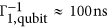during the readout sequence.

A probed TLS is characterized by the tunnelling energy Δp and the strain-dependent asymmetry energy εp (index p stands here for “probed”). In our experiments, we tune the asymmetry energy in-situ by slightly bending the sample chip using a piezo actuator23, resulting in εp(V) = ηp · (V − V0,p) where V is the applied piezo voltage and V0,p the voltage at which the probed TLS becomes symmetric. The coefficient ηp is given by ηp = γpϵ/∂V, where γp is the deformation potential which indicates how strongly the probed TLS couples to the applied strain. The strain is denoted by ϵ = δL/L and we estimate ∂ϵ/∂V ≈ 10−6/Volt based on results from a calibration of the piezo elongation per applied voltage and finite-elements-simulation of the mechanical chip deformation23. The TLS Hamiltonian reads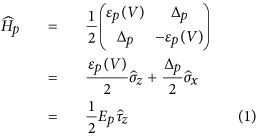with the Pauli matrices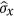and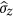. Diagonalization results in the energy difference between the TLS eigenstates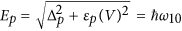, with Planck’s constant ħ and the TLS resonance frequency ω10. We defined the Pauli matrix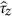in the eigenbasis of the TLS, which acts on the eigenstates as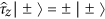.

## Experiment

To measure TLS decoherence rates as a function of their asymmetry energy, we first apply our swap spectroscopy method12 to obtain an overview of the TLS frequency distribution in the sample. We then select a TLS whose symmetry point lies in the experimentally accessible strain range and perform microwave spectroscopy to calibrate its resonance frequency as a function of strain (see Fig. 3a). From a hyperbolic fit, we obtain the TLS’ tunnelling energy Δp, its asymmetry εp(V) as a function of the applied piezo voltage, and the deformation potential γp. We then apply standard resonant microwave pulse sequences illustrated in the insets of Fig. 2 to observe the TLS’ coherent state evolution in the time domain13. After a calibration of the driving strength by observing Rabi oscillations (Fig. 2a), we measure the energy relaxation rate Γ1 ≡ 1/T1 by exciting the TLS with a π-pulse and fitting the decaying state population with an exponential exp(−Γ1 · t) as shown in Fig. 2b. The experimental results on Γ1 are summarized in Fig. 3b.Figure 3: Spectroscopy and results of decoherence measurements, obtained on four TLSs.

Next, we measure dephasing using the Ramsey (see Fig. 2c) and the spin-echo protocol (see Fig. 2d). In both of these protocols, the TLS is initalized into a superposition of the eigenstates using a π/2-pulse. The decay of this superposition assumes the general functional form exp[−Γ1t/2 − xi(t)], where i = R for Ramsey and i = E for spin-echo. The dephasing functions xR(t) and xE(t) depend strongly on the environment’s fluctuation spectrum and will be in the focus of our discussion below. Further details of these experiments are contained in Supplementary Information I.

Our time-domain data do not allow us to determine the exact functional form of the dephasing signal, because only a few oscillation periods are observed at asymmetry energies where Γφ,R dominates over Γ1 (see Fig. 2c). Since this renders fits to a linear dependence xi = Γφ,it to appear practically indistinguishable from a Gaussian decay xi = (Γφ,it)2, we estimate the pure dephasing rates Γφ,i in the linear approximation and deduce their functional form from their strain dependence. Figure set 3c summarizes the extracted effective dephasing times T2,R = (Γ1/2 + Γφ,R)−1 and T2,E = (Γ1/2 + Γφ,E)−1 of four different TLSs, which were measured in the same qubit sample. Using the previously obtained data on Γ1, we extract the pure dephasing rates Γφ,R and Γφ,E, which are shown in Fig. 3d. Additional data obtained from other TLSs are included in the Supplementary Information I.

### Energy relaxation

For all investigated TLSs, we observe (see Fig. 3b) that their energy relaxation rates exhibit a strain-dependent structure that appears symmetric with respect to the point of lowest TLS energy εp = 0. This indicates that the spectral density of the underlying relaxing modes depends only on frequency and is independent of the applied strain. Therefore, we conclude that the dominant relaxation mechanism of the probed TLSs is not due to their near-resonant coupling to other TLSs, because those would also be detuned by the applied strain and thus are expected to generate non-symmetric patterns in Γ1. This notion is further supported by the finding that strong mutual TLS interactions are rarely observed for our sample12.

If the noise spectral density was constant around ω = Δp/ħ, the strain dependence of Γ1 for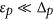would be given (see Supplementary Information II) by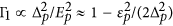, i.e. it would show a weak parabolic decrease around the symmetry point. As seen in Fig. 3b, such a scaling is obscured by the pronounced frequency-dependence of the noise spectral density. One may assume that this structure originates from the coupling to phonon modes which should have a discrete spectrum since the lateral size of the junction’s dielectric is comparable to the wavelength of high-frequency phonons. Indeed, a comparison of Γ1 of different TLS as a function of their resonance frequencies (see Supplementary Information I) reveals a common maximum at 7.4 GHz for 3 out of 5 investigated TLS, indicating that those TLS may be coupled to the same phononic mode24.

### Pure dephasing

The observed pure dephasing “rates” Γφ,R and Γφ,E show the following main features: a) the echo protocol is extraordinarily efficient, so that the ratios Γφ,Rφ,E reach very large values (see Table 1). b) the εp-dependence of the echo dephasing rate is clearly parabolic: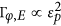; c) close to the symmetry point, the εp-dependence of the Ramsey dephasing rate Γφ,R could be fitted to a linear behavior, Γφ,R |εp|, in all TLSs. In the Supplementary Information II, we review shortly the well known results in order to identify possible sources of pure dephasing. We conclude that an environment characterized by white noise or by 1/f noise could not explain the experimental findings.

### Interpretation of the experimental results

We argue that the experimental observations can be explained in the framework of the standard tunnelling model20,21. In contrast to the probed high frequency TLS, whose energy splitting is much higher than the thermal energy,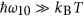, the TLSs responsible for pure dephasing are “thermal”, i.e. their energy splittings are lower than kBT so that they switch randomly between their states. We argue that the switching rates of thermal TLSs are very low, leading to the essentially non-Gaussian noise that has a spectral power more singular than 1/f. In this case, the Ramsey dephasing is dominated typically by the nearest neighbouring thermal TLS25,26,27. Since the asymmetry energies of the thermal TLSs also change with strain, one expects that some TLSs will go in and out of the group of relevant thermal TLSs as the strain is varied. Thus, the dominant decohering TLS will be replaced by another thermal TLS when the change of its asymmetry energy is on the order of the thermal energy kBT (that is, ~2π × 1 GHz). This gives rise to a non-regular behavior, reflected in a change of slope or small irregularities in the Ramsey dephasing rate as a function of strain as seen in Fig. 3d. Thus, we shall focus on a region of order |εp| < kBT ≈ 2π × 1 GHz (here and in other places we use ħ = 1) near the symmetry point and study the dephasing by a single thermal TLS. In this scenario, close to the symmetry point Γφ,R |εp| in the general case, or Γφ,R |εp|2 in the special case where the decohering TLS is near its own symmetry point. The dominant thermal TLS is almost completely eliminated by the echo protocol. This explains the very high efficiency of the echo technique. We argue that the echo dephasing rate due to the thermal TLSs is much lower than the one due to the residual white noise environment, which explains the observed.

### Theory

In the standard tunnelling model each isolated TLS is described by a Hamiltonian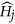as in Eq. (1), with the index p replaced by j. The asymmetry εj and tunneling energy Δj are assumed to be randomly distributed with a universal distribution function, where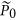is a material dependent constant15. Each TLS is also characterised by its coupling γj to the strain field. Moreover, it is well established that TLSs interact via phonon-mediated interactions, which can be described by a low-energy effective Hamiltonian of the form28,29,30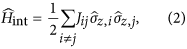with the interaction coefficients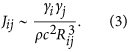Here Rij is the distance between the TLSs and ρ, c are the mass density and sound velocity, respectively. A central dimensionless parameter of the tunnelling model is the tunneling strength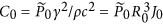, where R0 and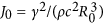are the typical distance and typical interaction strength between nearest neighbour TLSs, respectively. The well-known similarity in the low-temperature properties of disordered solids is reflected in a universal value of C0 ≈ 10−331,32.

We consider now the probed TLS interacting with a set of thermal TLSs via the coupling mechanism of Eq. (2). Only the coupling terms involving the slow (non-rotating) variables of both the probed TLS,, and of the thermal TLSs,, are relevant for pure dephasing. Moreover, at frequencies relevant for pure dephasing, the operatorscan safely be replaced by classical stochastic processes τz,j(t) describing random switching between τz,j = ±1 with switching rate Γ1,j. Thus, the Hamiltonian of the probed TLS reduces effectively to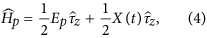where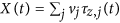. The effective couplings are given by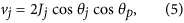where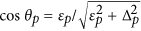and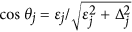. Here Jj is the coupling strength (3) between the probed TLS and thermal TLS number j.

The theory of pure dephasing due to a coupling to an ensemble of TLSs is discussed in Supplementary Information II. Here we provide the qualitative estimates. The effect of a thermal TLS on the coherence properties of the probed TLS depends on the coupling vj and on the switching rate (relaxation rate) Γ1,j of the thermal TLS. We assume that the random transitions of each TLS are mainly due to their coupling to phonons, in which case the relaxation rate reads21,33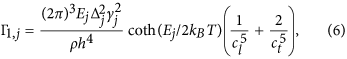where cl and ct are the sound velocities of the longitudinal and transverse modes, respectively. The maximum switching rate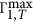among the thermal TLS for which Ej ≤ kBT (the TLSs with Ej > kBT are not thermal and do not give rise to low frequency noise) is obtained for Δj = Ej and Ej = kBT. Setting γj ≈ 1 eV and T = 35 mK10,15,23, we obtain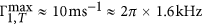.

Next, we estimate the typical coupling strength of the nearest thermal TLS, JT, by calculating the typical distance between the probed TLS and its nearest neighbouring thermal TLS in three and two dimensions (3D and 2D, respectively), and find (see Supplementary Information II)where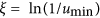, with umin being a lower cutoff for the parameter u ≡ sin2θ = (Δ/E)234, d ≈ 3 nm is the thickness of the tunnel dielectric, and we assumed the usual values C0 ≈ 10−3 and ξ ≈ 20.

The above estimates reveal that thermal TLSs satisfy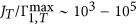. We recall the relation vj = Jj cos θj cos θp and take into account that, typically, cos θj = O(1). Thus, the closest thermal TLS is in the strong coupling regime,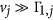, except in the very close vicinity of the symmetry point εp = 0 of the probed TLS. Therefore, we should study the εp-dependence of the dephasing rates assuming the presence of strongly coupled thermal TLSs. Such a situation also provides an explanation for the effectiveness of the echo protocol. This is clearly illustrated by an example of dephasing caused by a single TLS with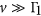(we drop the TLS index j) discussed in ref. 26. While the Ramsey dephasing “rate” is of order v, the echo dephasing “rate” is of order Γ1 of the thermal TLS (see Supplementary Information II for details). Thus, in this case, Γφ,R |εp|, whereas Γφ,E is independent of the applied strain.

The situation is more involved in the case of an ensemble of thermal TLSs. Since the coupling strength between the TLSs scales with their distance r as 1/r3, the closest thermal TLS dominates the Ramsey dephasing. Averaging the decay function over the distribution function of TLSs is not appropriate, i.e., there is no self-averaging (see Supplementary Information II). The typical Ramsey decay is approximately characterized by an envelope function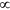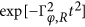, with possible few oscillations due to a small number of decohering TLSs26. The Ramsey dephasing “rate” reads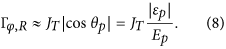In deriving Eq. (8), we assumed that the factor cos θj in Eq. (5) for the closest thermal TLS does not depend strongly on the applied strain. This assumption is valid in the most probable case, where the closest thermal TLS is not expected to be close to its own symmetry point (εj = 0) at the piezo voltage V = V0,p (i.e. at the same voltage for which the probed TLS is in its symmetry point). However, in the more special case in which the closest thermal TLS is near its symmetry point at V = V0,p, the Ramsey dephasing rate is expected to change quadratically with the piezo voltage, that is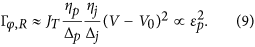This special situation could be of relevance for TLS1. Indeed, as one can observe in the leftmost column of Fig. 3d, a parabolic fitting could be performed here in a wider range of εp as compared to the shown linear fit.

For the echo decay due to a single strongly coupled thermal TLS, the dephasing rate is independent of εp. For an ensemble of TLSs it turns out that the decay function is not dominated by the closest TLS, but rather multiple TLSs contribute, i.e., there is self-averaging in this case (see Supplementary Information II). However, the theory predicts Γφ,E |εp|0.4 and Γφ,E |εp|0.5 in 2D and 3D, respectively, in disagreement with the experimental results that show a quadratic dependence of the echo dephasing rate. Moreover, the predicted order of magnitude is too small to explain the experimentally observed echo dephasing rate, i.e. the mechanism of interactions between TLSs is expected to yield echo efficiencies even stronger than those observed in our experiment. This is supported by the data obtained on TLS4 (see rightmost plot of Fig. 3d), for which the echo protocol is very efficient in the whole range of εp.

To explain the experimental findings we are forced to assume some extra white noise environment that leads (see Supplementary Information II) to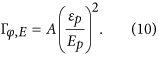Such a white noise environment could result e.g. from fast relaxing TLSs30 or from non-equilibrium quasiparticles35,36. Quasiparticles are well known to induce decoherence in superconducting quantum devices. An estimate of the dephasing rate induced by non-equilibrium quasiparticles is in good agreement with the fitting parameter A (see Supplementary Information II).

The above contribution of the white noise [Eq. (10)] gives a similar contribution to the Ramsey dephasing rate. Thus, combining (8) with (10) we attempt to fit Γφ,R in Fig. 3d in the vicinity of the symmetry point (|εp|/2π < 1 GHz) using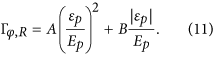As explained above, one should not expect a pure linear or parabolic behavior of the Ramsey dephasing rate on the whole range of εp. Being dominated by single thermal TLSs, the Ramsey dephasing rate is expected to exhibit a change of slope as the decohering TLSs go in and out of the set of thermal TLSs, that is when |εp|/2π > 1 GHz. Yet, in a typical case, one expects a linear behavior in a narrow vicinity of the symmetry point.

Table 1 summarizes the fitting parameters A and B as well as other extracted TLS parameters. According to our theory, the parameter B is associated with the coupling JT [Eqs (7) and (8)]. The estimations of the standard tunnelling model for JT in the 2D case are in good agreement with the fitting parameter B for all TLSs.

Summarizing, our measurements of TLS decoherence rates as a function of their asymmetry energy reveal that TLS relaxation occurs mainly due to their coupling to discrete phonon modes, while dephasing is dominated by their interaction with randomly fluctuating thermal TLS at low energies. Our theory predicts that thermal TLSs in the standard tunnelling model are characterized by, i.e. their coupling strength to the probed TLS exceeds their switching rate. Such TLSs produce noise which gives rise to an approximately linear dependence of the Ramsey dephasing rate of coherent TLS on the external strain (which, in more special cases, can also be quadratic). The Ramsey dephasing is dominated by a small number of thermal TLSs, which explains the observed irregularities in the Ramsey dephasing rate as a function of external strain. The order of magnitude of the measured Ramsey dephasing rate is in agreement with the theory. The strain dependence of the echo dephasing rate, on the other hand, can not be accounted for by the standard tunnelling model. Its explanation requires the presence of a white noise environment. This could consist e.g. of much faster fluctuators that are characterized by a weak interaction with the probed TLS, or non-equilibrium quasiparticles in the superconducting layers.

## Methods

The phase qubit sample used in this work was fabricated in the group of J. M. Martinis at University of California, Santa Barbara (UCSB), as described in ref. 37. The qubit junction had an area of about 1 μm2, fabricated using aluminum as electrode material and its thermally grown oxide as a tunnel barrier. All data have been obtained at a sample temperature of about 35 mK. The mechanical strain was controlled by bending the sample chip with a piezo transducer as explained in ref. 23.